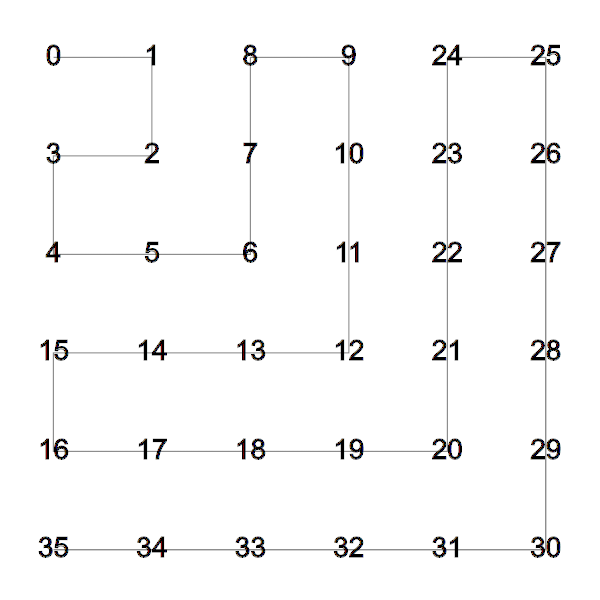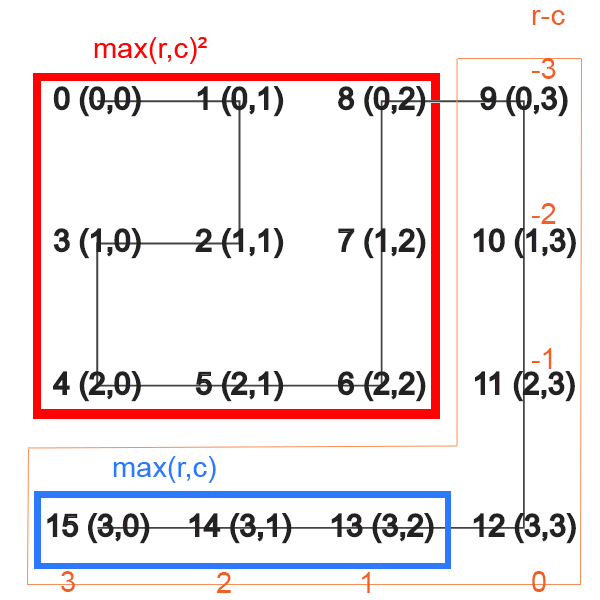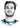# Enumerating a Grid in Spiral Order

Category:

I could only find complicated algorithms to enumerate a grid in a spiral manner. I’ll deduce a constant time one-liner in this article; if you only care about the code, skip all the explanation. We will iterate the grid in a spiral manner that is used, inter alia, by Cantor’s enumeration to prove that the rationals are countable.

## Spiral Enumeration

The algorithm will enumerate the grid in the following order starting at the top left:### The Algorithm

If r is the row, and c the column (0-based), then the following computes the index of the grid element (r,c) in the enumeration of the spiral:

``````var index = Math.pow(Math.max(r, c), 2) + Math.max(r, c)
+ (Math.max(r, c) % 2 ? 1 : -1) * (r - c);``````

### Explanation

The algorithm works layer by layer, where a layer is an L shaped piece that surrounds all the previous layers (which form a square). This sounds complicated, so let’s look at a picture:The third layer (0-based) is the orange L-shaped piece. There are 4 layers in the picture: 0, 1-3, 4-8, 9-15. The element (r,c) is in the `Math.max(r,c)`-st layer. Thus, the first index of this layer will be the “area” of the red square consisting of all the previous layers `Math.max(r,c)^2`. The enumeration inside the layer is given by `r-c` which are the orange numbers next to elements in the layer (the numbers from -3 to 3). But we want the first index in the layer to start at 0 instead of -3, so we shift everything by `Math.max(r,c)`, the layer’s width-1, represented in blue. The only thing left is to reverse the direction of odd layers, i.e., multiply `r-c` by `-1` when `Math.max(r,c) % 2 == 1`.

## The Inverse Mapping

This tells you how get the index in the spiral for an element (r,c) in the grid. But what if given an index in the spiral enumeration, you want to find the element in the grid position. It’s useful if you have a 2D grid and you want to access the elements in the order of the spiral enumeration, then you just iterate from `i=0..size` and compute the row and column `(r,c)` for each element. This requires finding the inverse mapping of the previous formula.

### The Algorithm

If `index` is the index of an element in the enumeration, `(r,c)` will be the element’s row and column.

``````var layer = Math.floor(Math.sqrt(index));
var indexInLayer = index - layer * (layer + 1);
var r = layer + Math.min(indexInLayer, 0);
var c = layer - Math.max(indexInLayer, 0);
if (layer % 2 == 0) {	// swap r and c
var tmp = r;
r = c;
c = tmp;
}``````

### Explanation

`Math.floor(Math.sqrt(index))` computes the layer for the index, and `layer * (layer + 1)` is the index of the bottom right element of that layer. Thus `index - layer * (layer+1)` is the index inside the layer, the orange numbers in the above picture. From then, we can compute the row `r` and the column `c` acting like we are in an odd layer. If we are indeed in an even layer, we just swap the row and column.

Both algorithms run in constant time and are quite simple to implement and understand. There are other ways to enumerate a grid in a spiral manner that are not covered here, for example you might not want to reverse the direction in odd layers. However, you should be able to apply the same techniques to find a formula for these different spiral orders.

# Hi, I'm Christoph Michel 👋I'm a , , and .

I mostly do and help small to mid-sized teams from all over the world 🌎 on both short and long-term projects on an independent contractor basis.

I strive for efficiency ⏱️ and therefore track many aspects of my life.